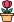엔지니어 게시판
LeetCode 솔루션 분류

# [11/18] 263. Ugly Number

### 작성자 정보

•작성
• 작성일

• 234 조회
• 1 댓글
• 0 추천
• 0 비추천

### 본문

Easy

An ugly number is a positive integer whose prime factors are limited to `2``3`, and `5`.

Given an integer `n`, return `true` if `n` is an ugly number.

Example 1:

```Input: n = 6
Output: true
Explanation: 6 = 2 × 3
```

Example 2:

```Input: n = 1
Output: true
Explanation: 1 has no prime factors, therefore all of its prime factors are limited to 2, 3, and 5.
```

Example 3:

```Input: n = 14
Output: false
Explanation: 14 is not ugly since it includes the prime factor 7.
```

Constraints:

• `-231 <= n <= 231 - 1`
Accepted
376,825
Submissions
889,626

댓글 1

## 학부유학생님의 댓글

• 익명
• 작성일
``````class Solution:
def isUgly(self, n: int) -> bool:

while n > 1:
if n % 2 == 0: n //= 2
elif n%3 == 0: n //= 3
elif n%5 == 0: n //= 5
else: break

return True if n == 1 else False``````
전체 349 / 1 페이지

• 등록일 00:48
• 등록일 02.01
• 등록일 02.01
• 등록일 02.01
• 등록일 02.01
• 등록일 02.01
• 등록일 02.01
• 등록일 02.01
• 등록일 02.01
• 등록일 02.01
• 등록일 02.01
• 등록일 02.01

• 등록일 01.31
• 등록일 01.31
• 등록일 01.31
• 등록일 01.27
• 등록일 01.27
• 등록일 01.23
• 등록일 01.23